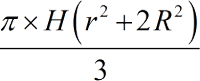Request a Tool

Barrel Volume Calculator

Calculate the volume of a barrel by given height and radii

Volume
0

Formula• π = a constant value 3.1415
• H = Height of the Barrel
• r = Middle radius of the Barrel
• R = Top Bottom radius of the Barrel

Defination / Uses

The barrel is approximately cylindrical in shape, with the exception of a bulge in the centre. The volume of a barrel is calculated using the barrel calculator. The supplied height and radius can be used to compute the volume of a barrel.

How barrel area calculator work?

Its a single step procedure.

• Add the required values in their corresponding fields.

Thats it! You will get your answer in less than a second. Use upper given formula in case of manual calculation.

To calculate the volume of a barrel, you'll need to know its height, top and bottom radiuses, and middle radius. This barrel volume calculator includes a major measurement units conversion function to get the output values in different customary units such as litres (L), gallons (gal), cubic inches (in), cubic foot (ft), cubic metres (m), and cubic centimetres (cm). For quick calculation use our weetools. No sign-up, registration OR captcha is required to use this tool.